Include your answers in this document in the sections below the rubric.

# AddHealth W4: Age at first intercourse vs pregancy

## Research question

Research question: Is there a relationship between having at least one pregancy (F = been pregnant, M = make partner pregnant) and the age at first vaginal intercourse for people in their fertile school years (12–22 years old)?

Intuition: The earlier you get started, the more opportunities you’ll have, and the more likely it will be.

## Data

Code book variables used to address this question. (Note, if you get in the habit of providing a personal codebook for your work you can give an analysis to someone else to read without having to explain it to them. Everything is documented (especially for your future self who doesn’t have as good a memory as you think).

ADDHEALTH: WAVE4 IN-HOME INTERVIEW CODE BOOK
Wave IV Section 15: Suicide, Sexual Experiences, & Sexually Transmitted Diseases

H4SE7 (AgeAtVag)
7. How old were you the first time you ever had vaginal intercourse?
1-30 years
96 refused
97 legitimate skip
98 don't know

H4TR9 (NPreg)
9. Thinking about all the relationships and sexual encounters you have ever
had, (how many times have you ever been pregnant/how many times have you ever
made a partner pregnant)? Include all pregnancies, whether they resulted in
babies born alive, stillbirth, abortion, miscarriage, or ectopic or tubal
pregnancy. [If Q.7=1] say: Be sure to include your current pregnancy in your
count.
1-19 pregancies
96 refused
98 don't know

Constructed variables
EverPreg = (NPreg > 0)

We create a data frame with nicely named variables, code and remove missing values, and code a binary variable for “ever pregnant” (EverPreg).

library(tidyverse)
## -- Attaching packages ----------------------------------- tidyverse 1.2.1 --
## v ggplot2 3.2.1     v purrr   0.3.2
## v tibble  2.1.3     v dplyr   0.8.3
## v tidyr   1.0.0     v stringr 1.4.0
## v readr   1.3.1     v forcats 0.4.0
## -- Conflicts -------------------------------------- tidyverse_conflicts() --
## x dplyr::lag()    masks stats::lag()
#library(PDS)
# NOTE:
#   if you don't have PDS installed,
#   and load it as described on UNM Learn / Resources / PDS Data:

# assign to a data frame
df.piv.preg <-
select(
h4se7
, h4tr9
) %>%
dplyr::rename(
AgeAtVag  = h4se7
, NPreg     = h4tr9
) %>%
# assign NA to missing values
mutate(
AgeAtVag  = replace(AgeAtVag, AgeAtVag %in% c(95), NA)
, NPreg     = replace(NPreg   , NPreg    %in% c(95), NA)
# set as a binary variable, if ever pregnant, then 1, otherwise 0
, EverPreg = (NPreg > 0)  # TRUE or FALSE (1 or 0)
# alternatively, to code as 1 and 0:
# EverPreg = ifelse(NPreg > 0, 1, 0)  # 1 or 0
) %>%
# remove NAs (the NAs break the logi.hist.plot() below)
na.omit() %>%
# keep people between 12 and 22 years old at first vaginal intercourse
filter(
AgeAtVag >= 12
, AgeAtVag <= 22
)

# plot histograms for each condition and fit a logistic curve
library(popbio)
logi.hist.plot(df.piv.preg$AgeAtVag, df.piv.preg$EverPreg
, logi.mod = 1 # logistic fit
, type = "hist", boxp = FALSE, rug = FALSE
, col = "gray"
, ylabel = "Probability at least one pregnancy (red)"
, ylabel2 = "Frequency"
, xlabel = "Age at first vaginal intercourse")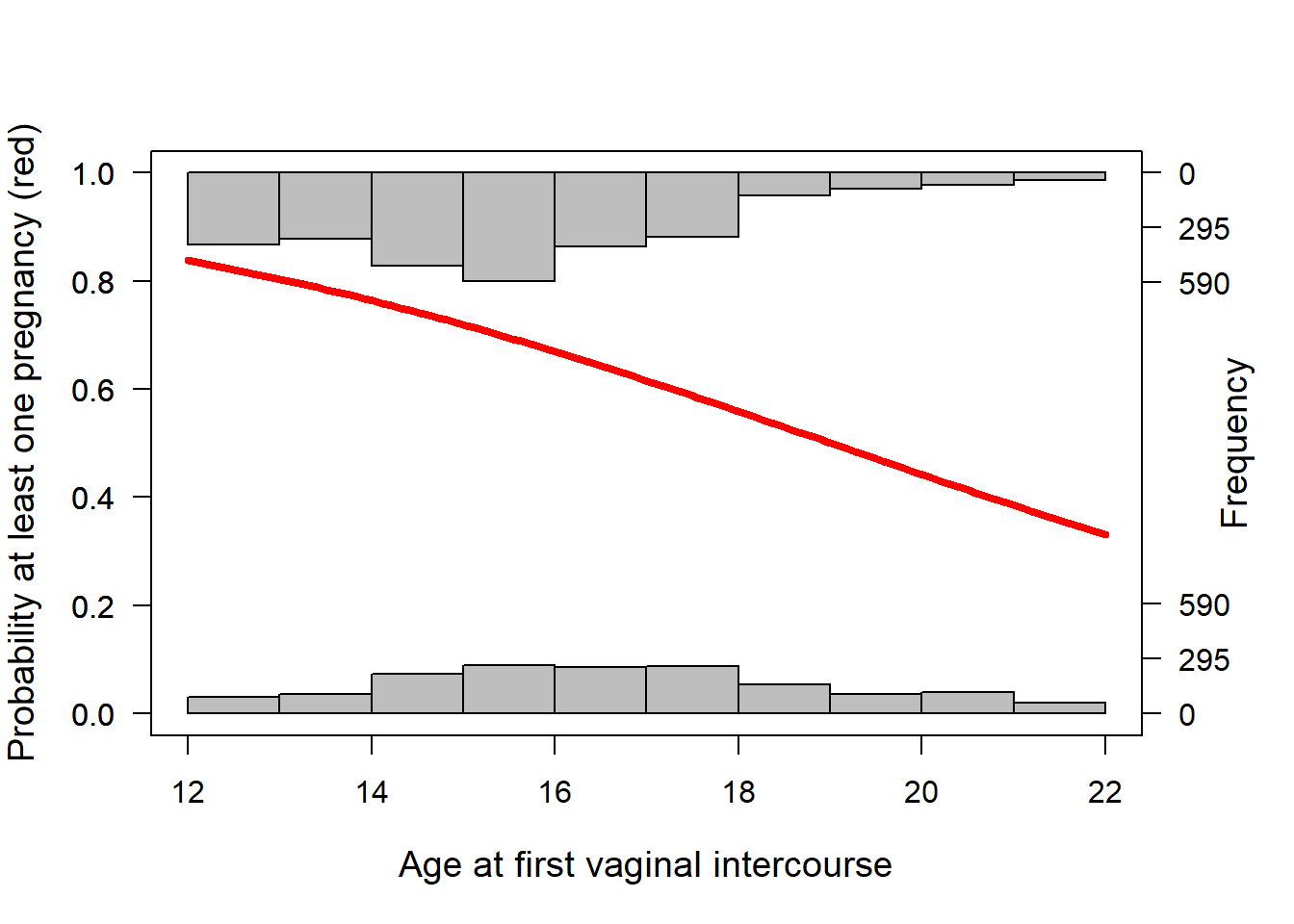Summarize observed probability of pregnancy for each age at first vaginal intercourse.

df.piv.preg.sum <- plyr::ddply(df.piv.preg, "AgeAtVag", summarise
, Total = length(EverPreg)
, Success = sum(EverPreg)
)
# create estimated proportion of preg for each age group
df.piv.preg.sum$p.hat <- df.piv.preg.sum$Success / df.piv.preg.sum$Total df.piv.preg.sum ## AgeAtVag Total Success p.hat ## 1 12 162 132 0.8148148 ## 2 13 311 254 0.8167203 ## 3 14 462 357 0.7727273 ## 4 15 719 503 0.6995828 ## 5 16 846 583 0.6891253 ## 6 17 648 396 0.6111111 ## 7 18 598 344 0.5752508 ## 8 19 278 123 0.4424460 ## 9 20 190 87 0.4578947 ## 10 21 179 65 0.3631285 ## 11 22 96 37 0.3854167 Plots on the probability scale should follow a sigmoidal curve (a little difficult to see here). Note that the overlayed reference curve (red) is a weighted smoothed curve (loess), not the model fit. library(ggplot2) p <- ggplot(df.piv.preg.sum, aes(x = AgeAtVag, y = p.hat)) p <- p + geom_point(aes(size = Total)) p <- p + geom_smooth(se = FALSE, colour = "red", aes(weight = Total)) # just for reference p <- p + expand_limits(y = 0) + expand_limits(y = 1) p <- p + labs(title = "Observed probability of at least one pregnancy") print(p) ## geom_smooth() using method = 'loess' and formula 'y ~ x'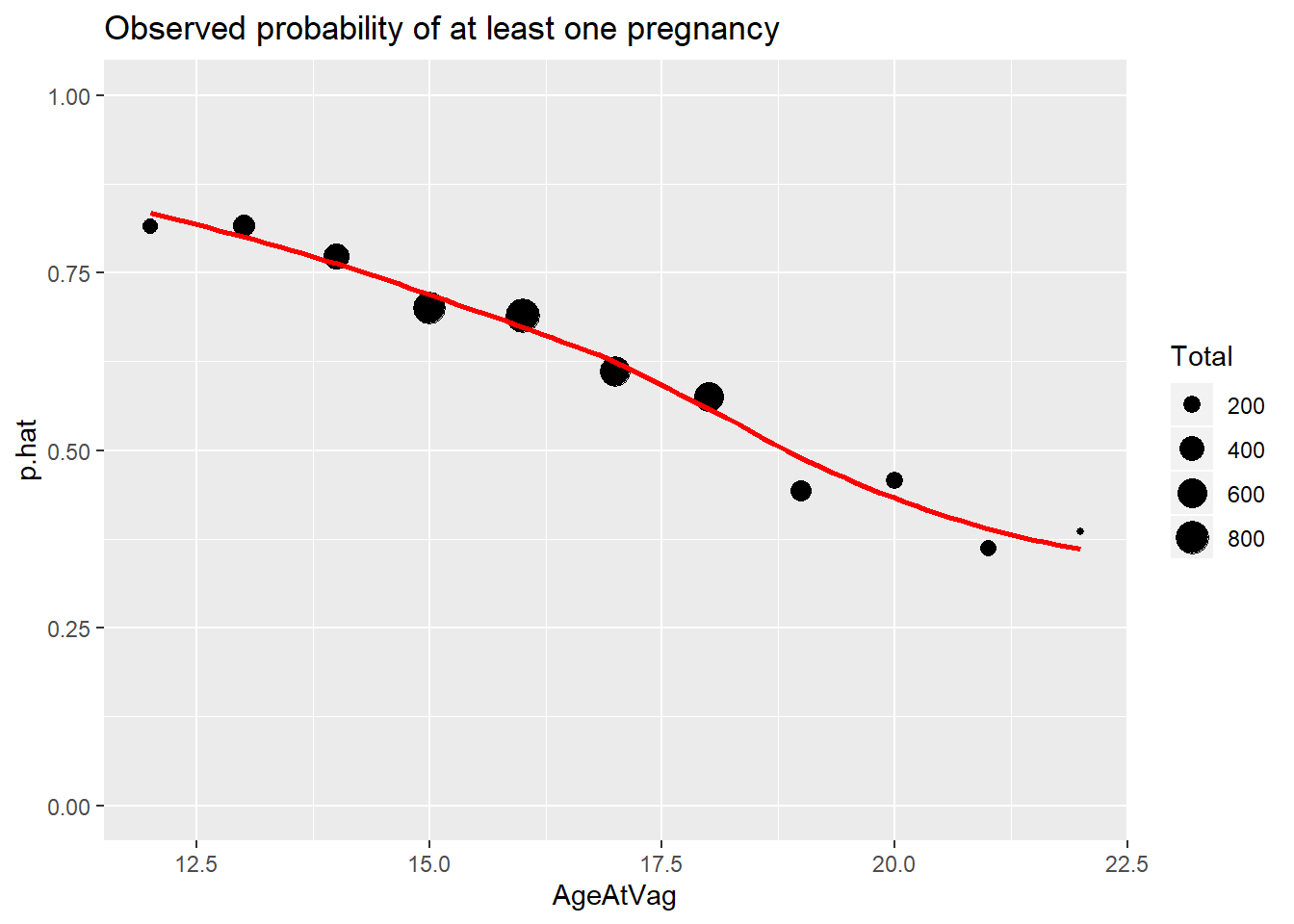On the logit scale, if points follow a straight line, then we can fit a simple linear logistic regression model. Note that the overlayed reference straight line (blue) is from weighted least squares (not the official fitted line), and the curve (red) is a weighted smoothed curve (loess). Both lines are weighted by the total number of observations that each point represents, so that points representing few observations don’t contribute as much as points representing many observations. # emperical logits df.piv.preg.sum$emp.logit <- log((    df.piv.preg.sum$p.hat + 0.5/df.piv.preg.sum$Total) /
(1 - df.piv.preg.sum$p.hat + 0.5/df.piv.preg.sum$Total))

library(ggplot2)
p <- ggplot(df.piv.preg.sum, aes(x = AgeAtVag, y = emp.logit))
p <- p + geom_point(aes(size = Total))
p <- p + stat_smooth(method = "lm", se = FALSE, aes(weight = Total))  # just for reference
p <- p + geom_smooth(se = FALSE, colour = "red", aes(weight = Total))  # just for reference
p <- p + labs(title = "Empirical logits")
print(p)
## geom_smooth() using method = 'loess' and formula 'y ~ x'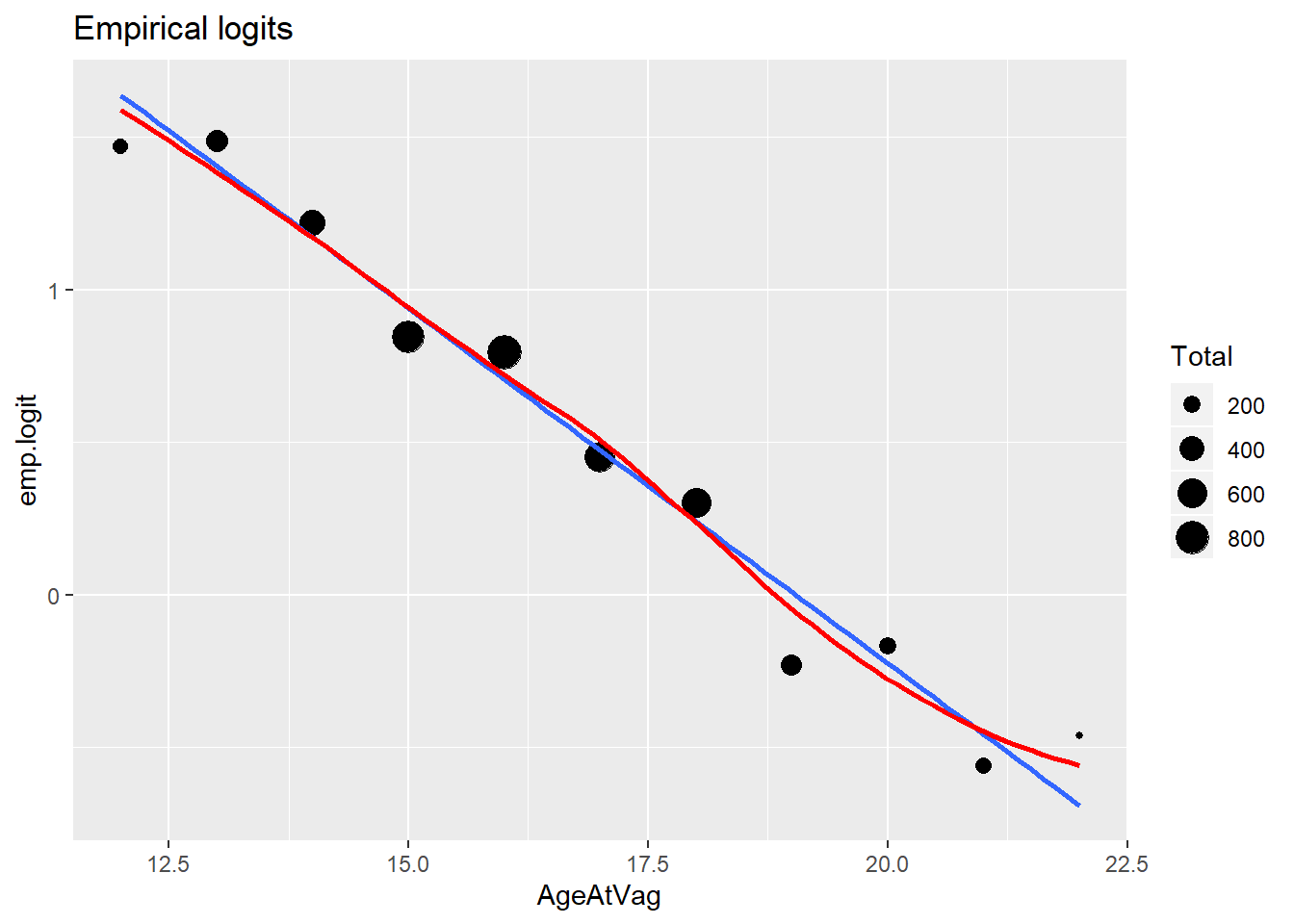(1 p) For the plot above, on the logit scale, does it appear that a straight line fits the data well? If not, what are the features in the data that aren’t being captured by the model?

## Simple logistic regression model

The simple logistic regression model expresses the population proportion $$p$$ of individuals with a given attribute (called the probability of success) as a function of a single predictor variable $$X$$. The model assumes that $$p$$ is related to $$X$$ through $\log \left( \frac{p}{1-p} \right) = \beta_0 + \beta_1 X$ or, equivalently, as $p = \frac{ \exp( \beta_0 + \beta_1 X ) }{ 1 + \exp( \beta_0 + \beta_1 X ) }.$ The logistic regression model is a binary response model, where the response for each case falls into one of two exclusive and exhaustive categories, success (cases with the attribute of interest) and failure (cases without the attribute of interest).

Fit the model.

# For our summarized data (with frequencies and totals for each age)
# The left-hand side of our formula binds two columns together with cbind():
#   the columns are the number of "successes" and "failures".
# For logistic regression with logit link we specify family = binomial,
#   where logit is the default link function for the binomial family.

# first-order linear model
glm.p.a <- glm(cbind(Success, Total - Success) ~ AgeAtVag, family = binomial, df.piv.preg.sum)

## Deviance statistic for lack-of-fit

Unfortunately, there aren’t many model diagnostics for logistic regression. One simple test is for lack-of-fit using the deviance. Under the null hypothesis (that you’ll state below), the residual deviance follows a $$\chi^2$$ distribution with the associated degrees of freedom.

Below we calculate the deviance p-value and perform the test for lack-of-fit.

# Test residual deviance for lack-of-fit (if > 0.10, little-to-no lack-of-fit)
glm.p.a$deviance ##  10.25947 glm.p.a$df.residual
##  9
dev.p.val <- 1 - pchisq(glm.p.a$deviance, glm.p.a$df.residual)
dev.p.val
##  0.329891

(1 p) State the null hypothesis for lack-of-fit.

(1 p) For your preferred model, the deviance statistic is $$D=???$$ with $$???$$ df. The p-value = ???.

(1 p) What is your conclusion for the model fit?

## Visualize and interpret the model

The glm() statement creates an object which we can use to create the fitted probabilities and 95% CIs for the population proportions at the ages at first vaginal intercourse. The fitted probabilities and the limits are stored in columns labeled fitted.values, fit.lower, and fit.upper, respectively.

Below I create confidence bands for the model and plot the fit against the data, first on the probability scale and then on the logit scale.

# put the fitted values in the data.frame
df.piv.preg.sum$fitted.values <- glm.p.a$fitted.values
pred <- predict(glm.p.a, data.frame(AgeAtVag = df.piv.preg.sum$AgeAtVag), type = "link", se.fit = TRUE) #$
df.piv.preg.sum$fit <- pred$fit
df.piv.preg.sum$se.fit <- pred$se.fit
# CI for fitted values
df.piv.preg.sum <- within(df.piv.preg.sum, {
fit.lower = exp(fit - 1.96 * se.fit) / (1 + exp(fit - 1.96 * se.fit))
fit.upper = exp(fit + 1.96 * se.fit) / (1 + exp(fit + 1.96 * se.fit))
})
# plot on probability scale
library(ggplot2)
p <- ggplot(df.piv.preg.sum, aes(x = AgeAtVag, y = p.hat))
# predicted curve and point-wise 95% CI
p <- p + geom_ribbon(aes(x = AgeAtVag, ymin = fit.lower, ymax = fit.upper), colour = "blue", linetype = 0, alpha = 0.2)
p <- p + geom_line(aes(x = AgeAtVag, y = fitted.values), colour = "blue", size = 1)
# fitted values
p <- p + geom_point(aes(y = fitted.values), colour = "blue", size=2)
# observed values
p <- p + geom_point(aes(size = Total), color = "black")
p <- p + expand_limits(y = 0) + expand_limits(y = 1)
p <- p + labs(title = "Observed and predicted pregnancy, probability scale")
print(p)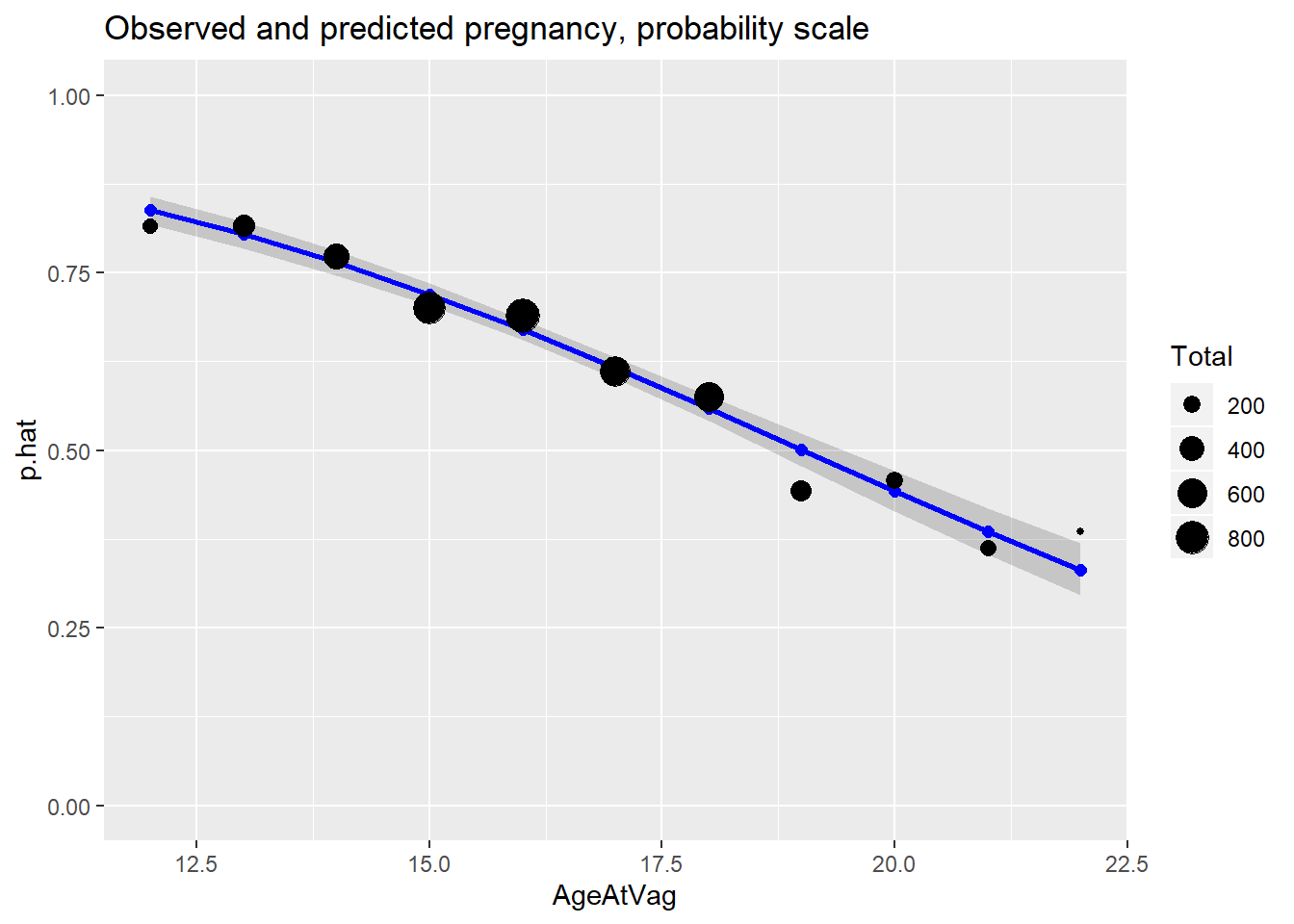(1 p) For the plot above, describe the general pattern of the probability of pregnancy given the age at first vaginal intercourse.

# plot on logit scale
library(ggplot2)
p <- ggplot(df.piv.preg.sum, aes(x = AgeAtVag, y = emp.logit))
# predicted curve and point-wise 95% CI
p <- p + geom_ribbon(aes(x = AgeAtVag, ymin = fit - 1.96 * se.fit, ymax = fit + 1.96 * se.fit), linetype = 0, alpha = 0.2)
p <- p + geom_line(aes(x = AgeAtVag, y = fit), colour = "blue", size = 1)
# fitted values
p <- p + geom_point(aes(y = fit), colour = "blue", size=2)
# observed values
p <- p + geom_point(aes(size = Total), color = "black")
p <- p + labs(title = "Observed and predicted pregnancy, logit scale")
print(p)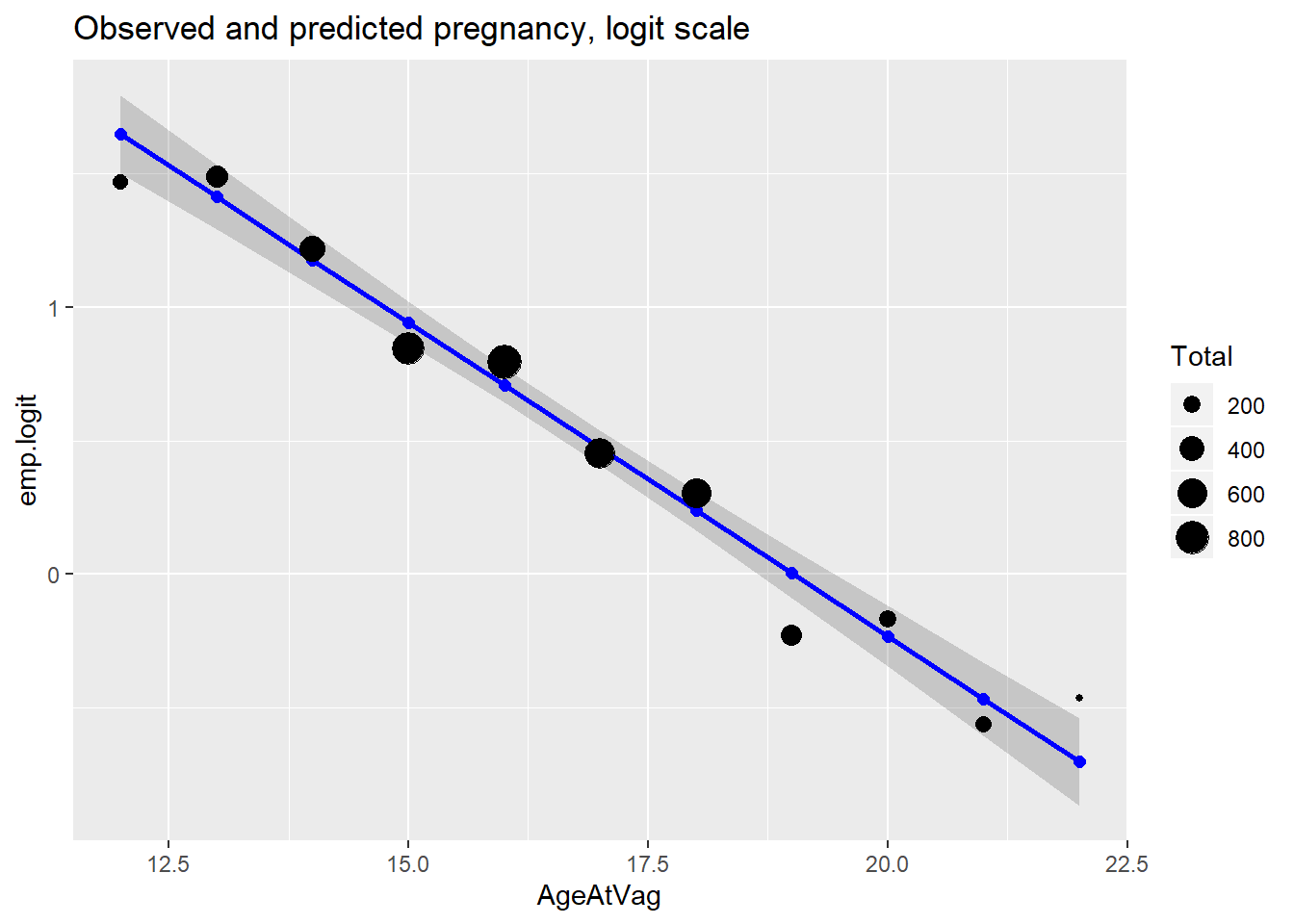(1 p) For the plot above, comment on the model fit.

Let’s consider those people who first had vaginal intercourse at age 15.

subset(df.piv.preg.sum, AgeAtVag == 15)
##   AgeAtVag Total Success     p.hat emp.logit fitted.values       fit
## 4       15   719     503 0.6995828 0.8439932      0.719588 0.9424188
##       se.fit fit.upper fit.lower
## 4 0.04010448 0.7351719 0.7034569

(1 p) Complete the statement below using r code in the sentence to automatically print the values.

“Using the model, the estimated population proportion of pregnancy when age at first intercourse was 15 is 0.72. We are 95% confident that the population proportion is between ??? and ???.”

The summary table gives MLEs and standard errors for the regression parameters. The z-value column is the parameter estimate divided by its standard error. The p-values are used to test whether the corresponding parameters of the logistic model are zero.

summary(glm.p.a)
##
## Call:
## glm(formula = cbind(Success, Total - Success) ~ AgeAtVag, family = binomial,
##     data = df.piv.preg.sum)
##
## Deviance Residuals:
##     Min       1Q   Median       3Q      Max
## -1.9504  -0.7104   0.4217   0.6775   1.1959
##
## Coefficients:
##             Estimate Std. Error z value Pr(>|z|)
## (Intercept)  4.46341    0.24274   18.39   <2e-16 ***
## AgeAtVag    -0.23473    0.01444  -16.25   <2e-16 ***
## ---
## Signif. codes:  0 '***' 0.001 '**' 0.01 '*' 0.05 '.' 0.1 ' ' 1
##
## (Dispersion parameter for binomial family taken to be 1)
##
##     Null deviance: 294.929  on 10  degrees of freedom
## Residual deviance:  10.259  on  9  degrees of freedom
## AIC: 81.104
##
## Number of Fisher Scoring iterations: 3
  # see names(summary(glm.p.a)) to find the object that has the coefficients.
# can also use coef(glm.p.a)

(1 p) Complete the equation below with the MLEs of the regression coefficients. The MLE of the predicted probabilities satisfy $\log \left( \frac{\hat{p}}{1-\hat{p}} \right) = ??? + ??? \textrm{ AgeAtVag}$

Interpreting the model coefficients is tricky because they’re on the logit scale. We’d prefer to think of them on the probability scale. We’ll cover other ways of interpretating these coefficients next semester. For now, I want you to interpret qualities of the slope and intercept.

(1 p) Interpret the sign ($$+$$ or $$-$$) of the slope.

(1 p) What is the interpretation of the intercept? Is it meaningful?